﻿ 瓦斯爆炸冲击波在变径管道中的传播特性

瓦斯爆炸冲击波在变径管道中的传播特性Propagation Characteristics of Gas Explosion Shock Waves in Variable Diameter Pipes

Abstract: In order to study the propagation characteristics of gas explosion shock wave in pipeline section, using ANSYS/LS-DYNA to establish the variable diameter pipe model, the gas mixture gas with a length of 0.4 m and a concentration of 9.5% is filled at the closed end of the pipe, and the propaga-tion characteristics of the gas explosion shock wave in the variable diameter pipe are simulated numerically. The velocity and overpressure of each measuring point at the pipe center and the pipe wall were measured. The results show that: The explosion wave from the gas explosion has a com-plex reflection and a reflux in the pipe diameter area, increased explosive intensity in the pipe di-ameter area. In the short time after the explosion wave passed through the diameter cross-section, there was a higher secondary overpressure peak, and the impact on the wall of the pipe was more serious. Therefore, in the development design of underground roadway, in order to deal with the possible gas explosion disaster, it is necessary to avoid sudden change of roadway area or slow down the variation degree of roadway area.

1. 引言

2. 数学模型

2.1. 运动方程

$\nu =\frac{\partial x\left(\xi ,t\right)}{\partial t}\text{\hspace{0.17em}}\text{\hspace{0.17em}}\omega ={\frac{\partial \xi \left(X,t\right)}{\partial t}|}_{X}$ (1)

2.2. 控制方程

2.2.1. 质量守恒方程

$M={\int }_{\Delta \xi }{\rho }_{\xi }\text{d}{\nu }_{\xi }={\int }_{\Delta x}{\rho }_{x}\text{d}{\nu }_{x}={\int }_{{\Delta }_{X}}{\rho }_{X}\text{d}{\nu }_{X}$ (2)

2.2.2. 动量守恒方程

${\frac{\partial }{\partial t}|}_{x}{\int }_{\Delta \xi }{\rho }_{\xi }{\nu }_{\xi }\text{d}{\nu }_{\xi }={\int }_{\partial \Delta \xi }{t}_{i}\text{d}{s}_{\xi }+{\int }_{\Delta \xi }{\rho }_{\xi }{f}_{i}\text{d}{\nu }_{\xi }$ (3)

2.2.3. 能量守恒方程

$E={V}_{sij}{\xi }_{ij}-\left(p+q\right)\stackrel{˙}{V}·$ (4)

3. 数值模拟

3.1. 模型的建立Figure 1. Pipe finite element model

3.2. 网格划分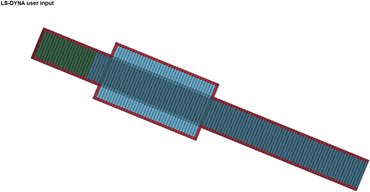Figure 2. Finite element model before and after meshing

3.3. 边界条件与初始条件

4. 模拟结果与分析

4.1. 瓦斯爆炸冲击波在整体管道内的传播特性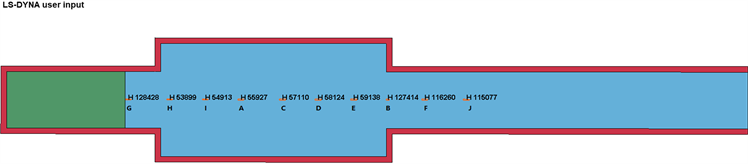Figure 3. In-pipe measuring points schematic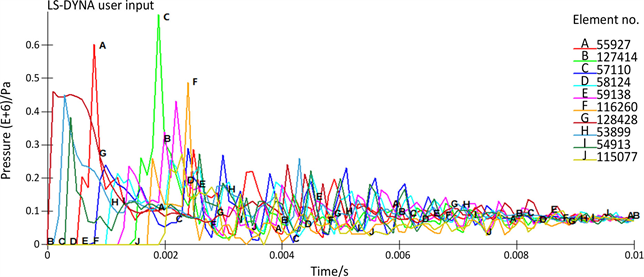Figure 4. Overpressure time history curve of each measuring point

4.2. 面积突扩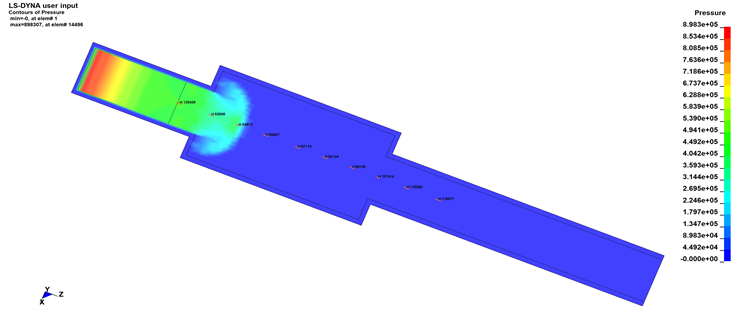(a) 0.0004 s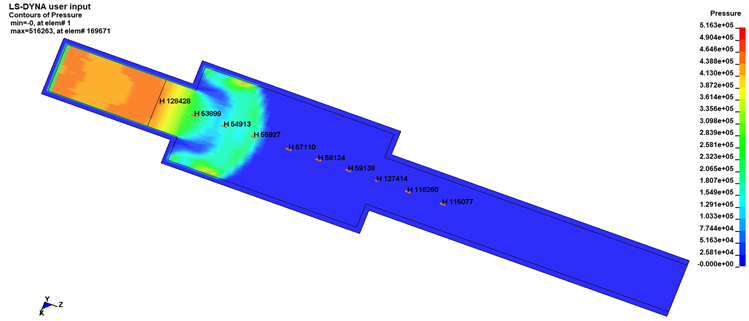(b) 0.0006 s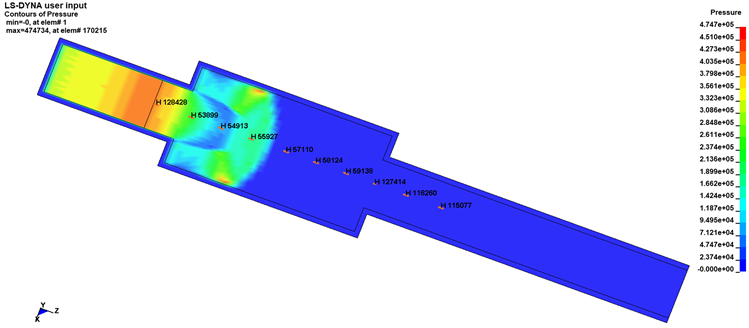(c) 0.0007 s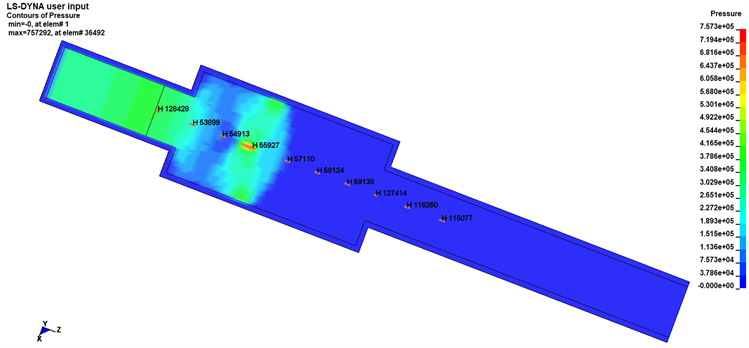(d) 0.0008 s

Figure 5. The pressure distribution after the explosion wave passes through the expanding area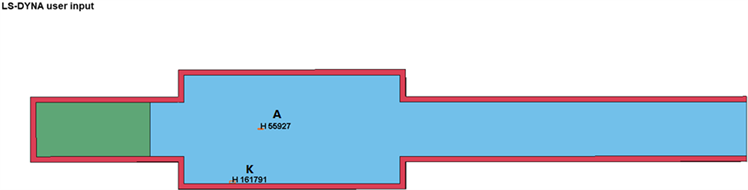Figure 6. Schematic of measuring point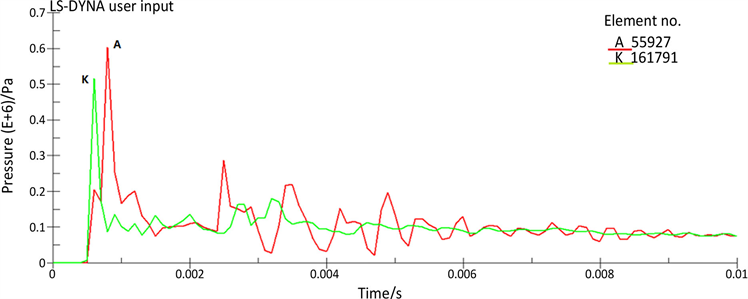Figure 7. A、K Measuring point overpressure time history curve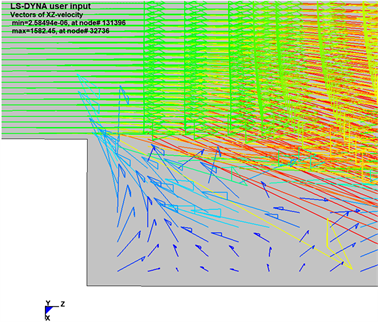Figure 8. Vector distribution of velocity in suddenly expanded area

4.3. 面积突缩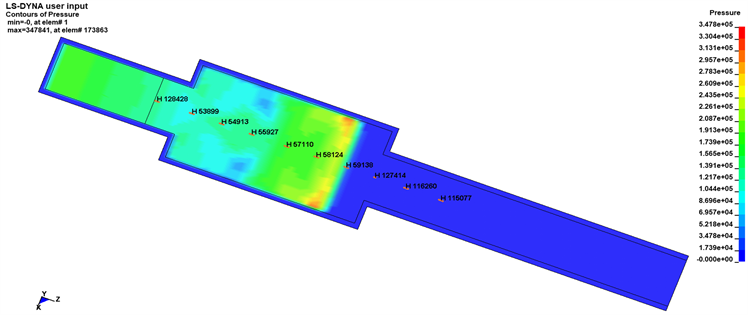(a) 0.0004 s(b) 0.0006 s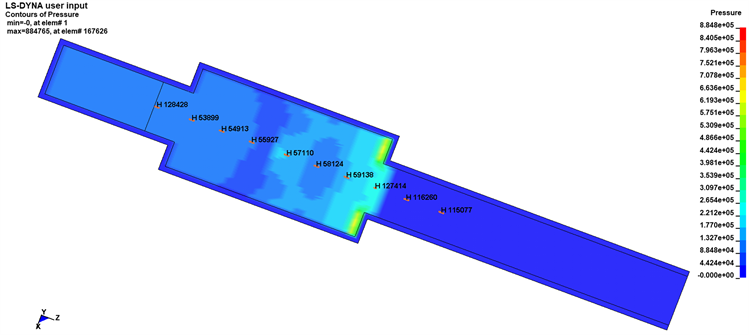(c) 0.0007 s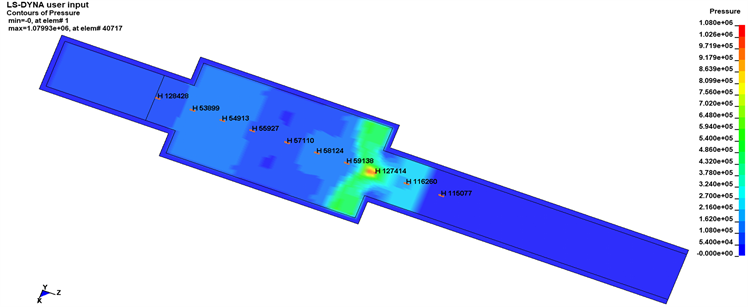(d) 0.0008 s

Figure 9. The pressure distribution after the explosion wave passes through the narrow area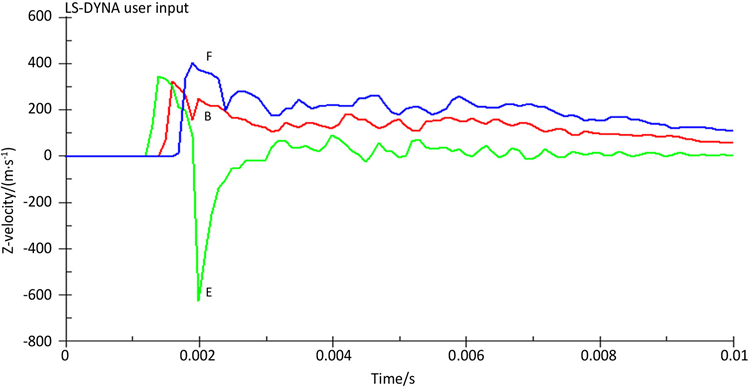Figure 10. Time-history curve of wave velocity in z directionFigure 11. Vector distribution of velocity in suddenly narrowing area

5. 结论

1) 变径管道内发生瓦斯爆炸后，在管道变径区域的反流现象和爆炸波复杂反射的共同作用，导致变径截面区域测点出现了更高的二次超压峰值。

2) 对比规则的等截面直管，变径管道中的瓦斯爆炸传播过程更加复杂，爆炸波波速和峰值超压都要远大于规则管道，对管道壁面的冲击更加严重。

3) 在矿井井下，尤其是易发生瓦斯爆炸的区域，应尽量避免巷道截面面积的突变，或者减小截面面积的变化程度，并利用面积突变巷道瓦斯爆炸传播规律采取对应举措，来降低瓦斯爆炸所带来的损失。

 Jia, Z.Z., Zheng, C.S., et al. (2018) Similarity Simulation of Mining-Crack-Evolution Characteristics of Overburden Strata in Deep Coal Mining with Large Dip. Journal of Petroleum Science and Engineering, 165, 477-487.
https://doi.org/10.1016/j.petrol.2018.02.044

 Ye, Q., Jia, Z.Z. and Zheng, C.S. (2017) Study on Hydrau-lic-Controlled Blasting Technology for Pressure Relief and Permeability Improvement in a Deep Hole. Journal of Pe-troleum Science and Engineering, 159, 433-442.
https://doi.org/10.1016/j.petrol.2017.09.045

 Ye, Q., Wang, W.J., et al. (2017) Numerical Simulation on Tendency Mining-Fracture-Evolution Characteristics of Overlying Strata and Coal Seams above Working Face with Large Inclination Angle and Mining Depth. Arabian Journal of Geosciences, 10, 1-15.
https://doi.org/10.1007/s12517-017-2856-9

 皮子坤, 贾宝山, 贾廷贵, 等. 基于前景理论和区间数的煤矿瓦斯爆炸风险评价[J]. 中国安全科学学报, 2017, 27(6): 91-96.

 李润求, 施式亮, 念其锋, 等. 近10年我国煤矿瓦斯灾害事故规律研究[J]. 中国安全科学学报, 2011, 21(9): 143-151.

 徐景德. 矿井瓦斯爆炸冲击波传播规律及影响因素的研究[D]: [硕士学位论文]. 北京: 中国矿业大学, 2003.

 薛景宏, 储阳华. 温度作用下变径管道的应力应变分析[J]. 重庆理工大学学报(自然科学), 2017, 31(8): 51-56.

 景国勋, 贾智伟, 杨书召. 巷道截面积突变处瓦斯爆炸冲击波传播规律理论研究[J]. 科技导报, 2008(11): 62-64.

 印华融, 翁春生. 爆轰波在变截面突扩管道中的传播特性研究[J]. 航空兵器, 2016(6): 66-72.

 蔺照东, 李如江, 陈兴, 王等. 水平管道截面积突然扩大对冲击波传播的影响[J]. 煤矿安全, 2014, 45(5): 141-143+147.

 尤明伟, 傅伟斌, 蒋军成. 连通容器气体密闭爆炸与泄爆过程的实验研究[J]. 中国安全生产科学技术, 2014, 10(7): 11-15.

 姚世琪. 连通容器内可燃气体爆炸影响因素的数值分析[D]: [硕士学位论文]. 大连: 大连理工大学, 2011.

 黄佩玉. 连通容器内可燃气体爆炸及泄爆过程的数值模拟[D]: [硕士学位论文]. 赣州: 江西理工大学, 2015.

 江丙友, 林柏泉, 朱传杰, 翟成, 李子文. 瓦斯爆炸冲击波在并联巷道中传播特性的数值模拟[J]. 燃烧科学与技术, 2011, 17(3): 250-254.

 王海燕, 曹涛, 周心权, 谭国庆, 谢建国, 姜伟. 煤矿瓦斯爆炸冲击波衰减规律研究与应用[J]. 煤炭学报, 2009, 34(6): 778-782.

 曲志明, 周心权, 王海燕, 马洪亮. 瓦斯爆炸冲击波超压的衰减规律[J]. 煤炭学报, 2008(4): 410-414.

 Ye, Q., et al. (2017) Experimental Study on the Influence of Wall Heat Effect on Gas Explosion and Its Propagation. Applied Thermal Engineering, 118, 392-397.
https://doi.org/10.1016/j.applthermaleng.2017.02.084

 赵丹, 齐昊, 潘竞涛, 等. 不同类型管道内瓦斯爆炸冲击波传播试验研究[J]. 中国安全科学学报, 2018, 28(3): 79-83.

 林柏泉, 叶青, 翟成, 菅从光. 瓦斯爆炸在分岔管道中的传播规律及分析[J]. 煤炭学报, 2008(2): 136-139.

 吕祥锋, 潘一山, 李忠华, 代树红. 冲击波作用下巷道破坏规律相似模拟研究[J]. 振动与冲击, 2011, 30(10): 212-215 + 235.

 Alia, A. and Souli, M. (2006) High Explosive Simulation Using Multi-Material Formulations. Applied Thermal Engineering, 26, 1032-1042.
https://doi.org/10.1016/j.applthermaleng.2005.10.018

 张雄, 陆明万, 王建军. 任意拉格朗日-欧拉描述法研究进展[J]. 计算力学学报, 1997(1): 93-104.

 吴燕燕. 室内燃气爆炸作用下钢框架结构动力响应与连续倒塌分析[D]: [硕士学位论文]. 哈尔滨: 东北林业大学, 2012.

 石少卿, 康建功, 江敏, 等. ANSYS/LS-DYNA在爆炸与冲击领域内的工程应用[M]. 北京: 中国建筑工业出版社, 2013: 44-46.

 菅从光, 林柏泉, 翟成. 瓦斯爆炸过程中爆炸波的结构变化规律[J]. 中国矿业大学学报, 2003(4): 25-28.

 叶青, 林柏泉. 受限空间瓦斯爆炸传播特性[M]. 徐州: 中国矿业大学出版社, 2012: 80-81.

Top